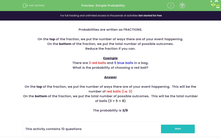# Simple Probability

In this worksheet, students work out the probability of an event happening.Key stage:  KS 3

Curriculum topic:   Probability

Curriculum subtopic:   Record, Describe and Analyse Probability Experiments

Difficulty level:#### Worksheet Overview

Probabilities are written as FRACTIONS.

On the top of the fraction, we put the number of ways there are of your event happening.

On the bottom of the fraction, we put the total number of possible outcomes.

Reduce the fraction if you can.

Example

There are 3 red balls and 5 blue balls in a bag.

What is the probability of choosing a red ball?

On the top of the fraction, we put the number of ways there are of your event happening.  This will be the number of red balls (i.e. 3)

On the bottom of the fraction, we put the total number of possible outcomes.  This will be the total number of balls (3 + 5 = 8)

The probability is 3/8

### What is EdPlace?

We're your National Curriculum aligned online education content provider helping each child succeed in English, maths and science from year 1 to GCSE. With an EdPlace account you’ll be able to track and measure progress, helping each child achieve their best. We build confidence and attainment by personalising each child’s learning at a level that suits them.

Get started